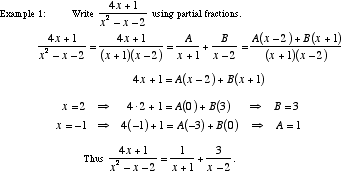index: click on a letter A B C D E F G H I J K L M N O P Q R S T U V W X Y Z A to Z index index: subject areas numbers & symbols sets, logic, proofs geometry algebra trigonometry advanced algebra & pre-calculus calculus advanced topics probability & statistics real world applications multimedia entrieswww.mathwords.com about mathwords website feedback

 Partial Fractions The process of writing any proper rational expression as a sum of proper rational expressions. This method is use in integration as shown below. Note: Improper rational expressions can also be rewritten using partial fractions. You must, however, use polynomial long division first before finding a partial fractions representation.See also Integration methods